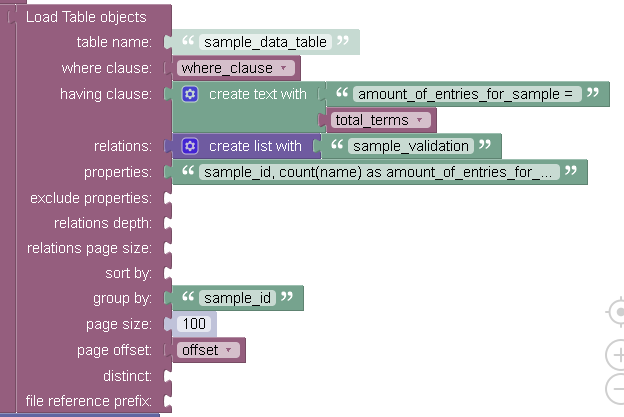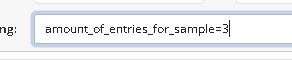# Query with relationship returning 0 rows when it should return more

I have a table with a 1 to1 relationship to another table.

my load table objects looks like thisthe relations “sample_validation” is a column in table sample_data_table which has a one to one relationship with table “sample_validation_table” (which has a column in it called proximate_validation )

the where clause is :

``````( name='al' AND value<'2000') or  ( name='ca' AND value<'600') or  (sample_validation.proximate_validation in ('Acceptable'))
``````

if I run this where clause without the last section

``````( name='al' AND value<'2000') or  ( name='ca' AND value<'600')
``````

it returns 4 records (exactly as expected ) adding on the last part should have also returned 4 records because the proximate_validation column in table sample_validation_table is “Acceptable” of each of these records.

so this is my first attempt at pulling related data in a query. I did take a look at ( https://www.youtube.com/watch?v=KTCMWHI7A5E ) and tried to model my query with it.
I’m not sure what I’m doing wrong.
any thoughts would be really appreciated.
H

I recommend recreating your query in the `REST Console` on the `Data` screen and making sure you get data. Once it is done, it will be easier to transform it into a request in Codeless.

after looking at it in the rest console I think the issue is with my “having” clause

in my properties of the load table objects i have “sample_id, count(name) as amount_of_entries_for_sample”

but the relationship column is sample_validation.proximate_validation so its not being counted with the count function.

since my having clause is based on name count its too high and I get no results.

I tried adding “sample_validation.proximate_validation as name” to the properties in my code but that failed and I did not see a way to try this with the rest console

The `having clause` applies when you use an aggregating function. Do you use it?

here’s the documentation:
https://backendless.com/docs/rest/data_data_filtering.html

yes. in rest console (and in the codeless )

in rest it looks like this: (this part works as expected )

but I need to include the results from the relationship
so my query looks like this

( name=‘al’ AND value<‘2000’) or ( name=‘ca’ AND value<‘600’) or (sample_validation.proximate_validation=‘Acceptable’)

but when I changeI get no results because sample_validation.proximate_validation is not “name” which is the item being counted, so there are no items meeting the having clause.

I wanted to try ‘sample_validation.proximate_validation as name’ but there is no place I could find in the rest console to do that.

That’s not what the screenshot shows. Does it work with that where clause?

here is the results for the quarry

``````( name='al' AND value<'2000') or  ( name='ca' AND value<'600') or  (sample_validation.proximate_validation='Acceptable')
``````

it works (no errors) but I get no rows back which should not be the case as I know sample_validation.proximate_validation = Acceptable for the 4 samples returned with the original query

``````( name='al' AND value<'2000') or  ( name='ca' AND value<'600')
``````

I believe this is because sample_validation.proximate_validation is not included in the count(name) function.

The way it works is the initial data set is formed based on the where clause, then the aggregation kicks in and some data will be eliminated with the `having clause`. It looks like all the data is eliminated thus resulting in an empty response.

I agree that is the problem. there are 3 terms
( name=‘al’ AND value<‘2000’)
( name=‘ca’ AND value<‘600’)
(sample_validation.proximate_validation=‘Acceptable’)

oh! I think I just figured it out.

( name=‘al’ AND value<‘2000’ AND sample_validation.proximate_validation=‘Acceptable’) or ( name=‘ca’ AND value<‘600’ AND sample_validation.proximate_validation=‘Acceptable’)

I need to test this with more edge cases. I’ll update either way.

H

This is the solution.

I have table A and table B a relationship from A to B would be many to one. But if I went from B to A it would be a one to one (I saw this in one of your video’s ) So thats what I did and it made things so much simpler.

columns Table B (sample_id, elementname,value, sample_validationone:one:relationship with table A )
columns Table A (sample_id, proximate_validation)

a query to table B will return multiple examples for a sample_id (there will be 75 “elementname” and value pairs for each sample_id ) but the relationship sample_validationone points to the same sample_id entry in Table A so it was just a matter of adding the relationship query to each term in the main query.

``````     query term 1                                 query term2                           .... query term N
``````

( name=‘al’ AND value<‘2000’ ) or ( name=‘ca’ AND value<‘600’’)

to

( name=‘al’ AND value<‘2000’ AND sample_validation.proximate_validation=‘Acceptable’) or ( name=‘ca’ AND value<‘600’ AND sample_validation.proximate_validation=‘Acceptable’)

and everything works. This is only possible because saw your video on how you can reverse a one to many into a one:one relationship.

Thanks for the help, sometimes someone else’s perspective can help you see a problem in a different way.
H